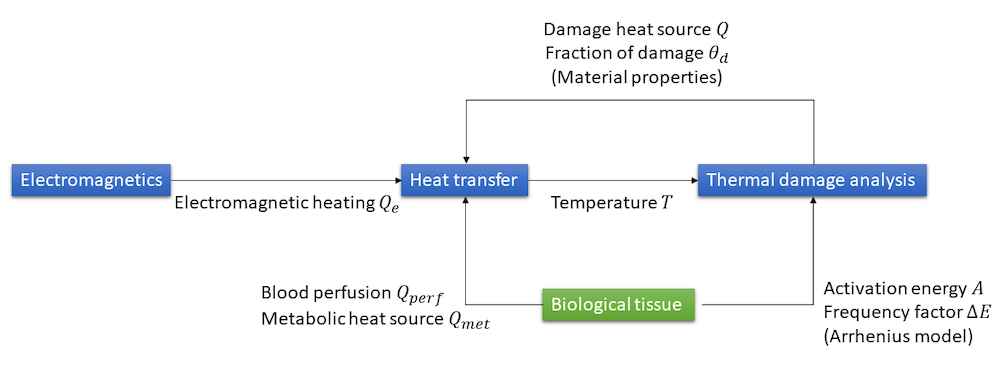# 生物组织中的传热与热损伤分析

2019年 11月 21日

### 癌症热疗法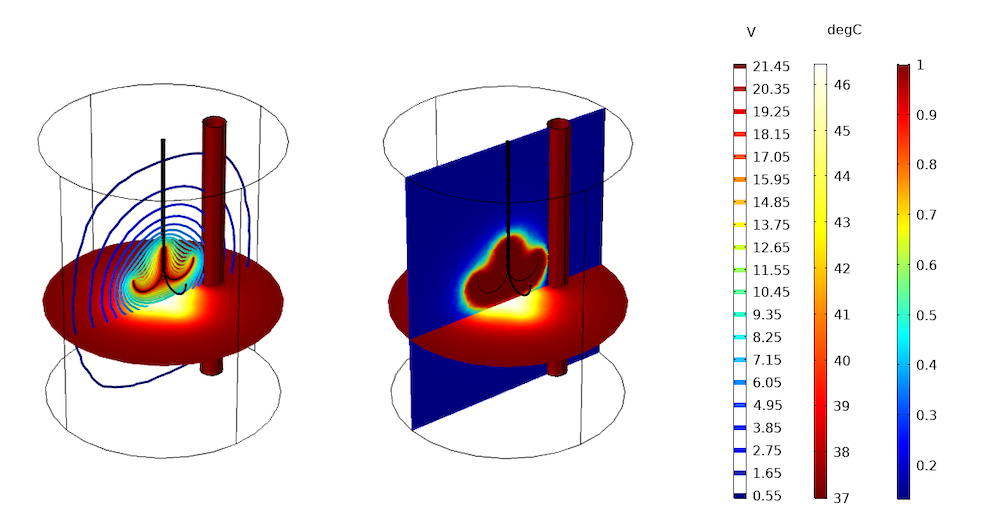### 生物组织中的传热分析

\rho C_p\frac{dT} {dt}-\nabla\cdot\left(k\nabla T\right) = \rho_\textrm{b}C_{p,\textrm{b}}\omega_\textrm{b}(T_\textrm{b}-T) + Q_\textrm{e}+ Q_\textrm{met}

C_{p,\textrm{b}}\omega_\textrm{b}(T_\textrm{b}-T)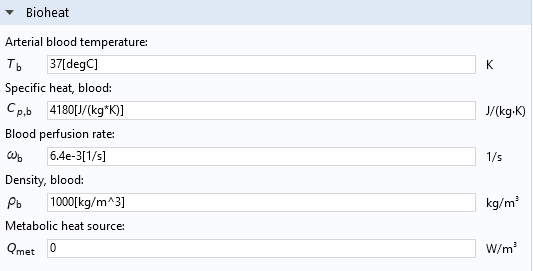{e}
，并考虑了电磁表面损耗，其值取决于加热设备的类型。Q_{e} 的特定计算表达式由具体的电磁物理场接口决定，包括焦耳热激光加热感应加热微波加热预定义物理接口。

### 计算生物组织的热损伤

#### 温度阈值模型1. 当组织温度超过损伤温度 T_\textrm{d,h}，大于时间 t_\textrm{d,h}
2. 组织温度刚超过坏死温度 T_\textrm{n,h}

\alpha(t)=\alpha_0+\frac{1}{t_\textrm{d,h}}\int_0^t(T>T_\textrm{d,h})dt

#### 阿累尼乌斯动力学模型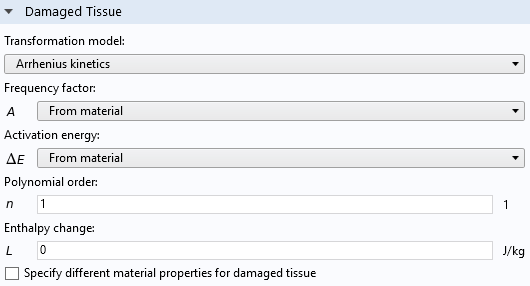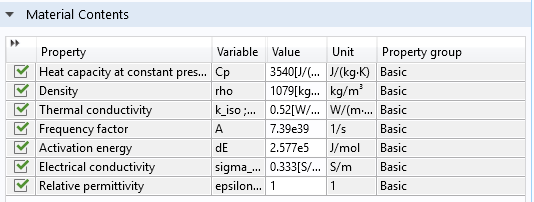\alpha(t)=\alpha_0+\int_0^t(1-\alpha)^nAe^{\frac{-\Delta E}{RT}}dt

#### 预测组织的损伤程度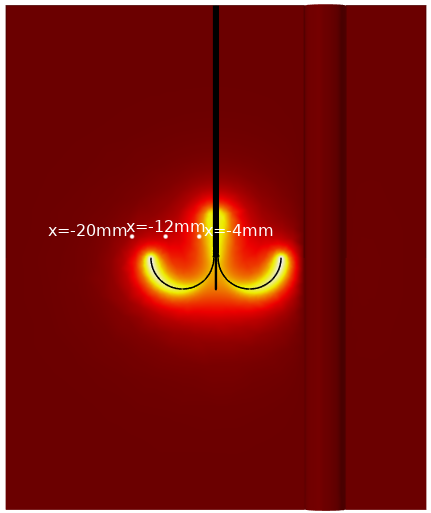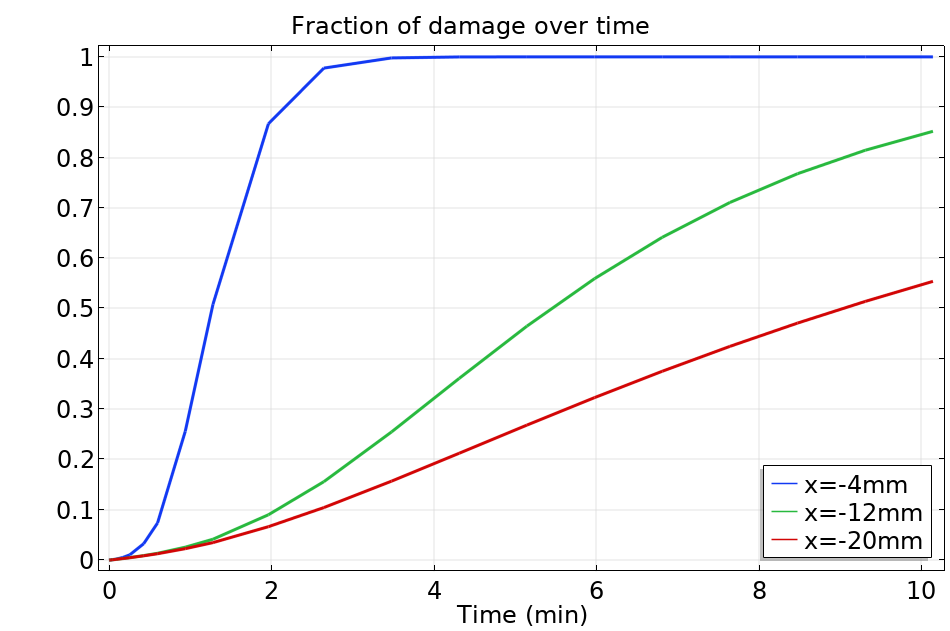#### 指定受损组织的材料属性，处理受损热源

k=\theta_\textrm{d}k_\textrm{damaged}+(1-\theta_\textrm{d})k_\textrm{healthy}

### 结语